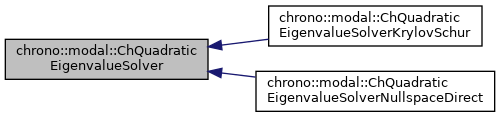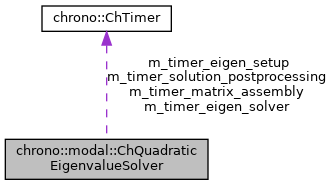## Description

Base interface class for eigensolvers for the damped dynamic problem ie.

the quadratic eigenvalue problem (lambda^2*M + lambda*R + K)*x = 0 also (-w^2*M + i*w*R + K)*x = 0, with complex w (where w.length() = undamped nat.freq) Children classes can implement this in different ways, overridding Solve()

#include <ChEigenvalueSolver.h>[legend][legend]

## Public Member Functions

virtual bool Solve (const ChSparseMatrix &M, const ChSparseMatrix &R, const ChSparseMatrix &K, const ChSparseMatrix &Cq, ChMatrixDynamic< std::complex< double >> &V, ChVectorDynamic< std::complex< double >> &eig, ChVectorDynamic< double > &freq, ChVectorDynamic< double > &damping_ratio, ChEigenvalueSolverSettings settings=0) const =0
Solve the quadratic eigenvalue problem (lambda^2*M + lambda*R + K)*x = 0 s.t. More...

double GetTimeMatrixAssembly () const
Get cumulative time for matrix assembly.

double GetTimeEigenSetup () const
Get cumulative time eigensolver setup.

double GetTimeEigenSolver () const
Get cumulative time eigensolver solution.

double GetTimeSolutionPostProcessing () const
Get cumulative time for post-solver solution postprocessing.

## Protected Attributes

ChTimer m_timer_matrix_assembly
timer for matrix assembly

ChTimer m_timer_eigen_setup
timer for eigensolver setup

ChTimer m_timer_eigen_solver
timer for eigensolver solution

ChTimer m_timer_solution_postprocessing
timer for conversion of eigensolver solution

## ◆ Solve()

 virtual bool chrono::modal::ChQuadraticEigenvalueSolver::Solve ( const ChSparseMatrix & M, const ChSparseMatrix & R, const ChSparseMatrix & K, const ChSparseMatrix & Cq, ChMatrixDynamic< std::complex< double >> & V, ChVectorDynamic< std::complex< double >> & eig, ChVectorDynamic< double > & freq, ChVectorDynamic< double > & damping_ratio, ChEigenvalueSolverSettings settings = 0 ) const
pure virtual

Solve the quadratic eigenvalue problem (lambda^2*M + lambda*R + K)*x = 0 s.t.

Cq*x = 0 If n_modes=0, return all eigenvalues, otherwise only the first lower n_modes.

Parameters
 M input M matrix R input R matrix K input K matrix Cq input Cq matrix of constraint jacobians V output matrix with eigenvectors as columns, will be resized eig output vector with eigenvalues (real part not zero if some damping), will be resized freq output vector with n undamped frequencies [Hz], as f=w/(2*PI), will be resized. damping_ratio output vector with n damping rations r=damping/critical_damping. settings optional: settings for the solver, or n. of desired lower eigenvalues. If =0, return all eigenvalues.

The documentation for this class was generated from the following file:
• /builds/uwsbel/chrono/src/chrono_modal/ChEigenvalueSolver.h